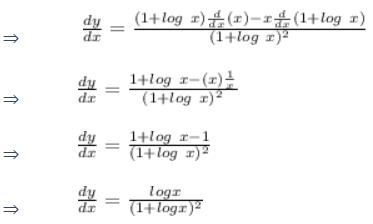# Test: Logarithmic Functions

## 10 Questions MCQ Test Mathematics (Maths) Class 12 | Test: Logarithmic Functions

Description
This mock test of Test: Logarithmic Functions for JEE helps you for every JEE entrance exam. This contains 10 Multiple Choice Questions for JEE Test: Logarithmic Functions (mcq) to study with solutions a complete question bank. The solved questions answers in this Test: Logarithmic Functions quiz give you a good mix of easy questions and tough questions. JEE students definitely take this Test: Logarithmic Functions exercise for a better result in the exam. You can find other Test: Logarithmic Functions extra questions, long questions & short questions for JEE on EduRev as well by searching above.
QUESTION: 1

### The differential coefficient  dy/dx  of the function yx = xy

Solution:

d(xy)=d(e(y⋅log(x)))
=e^(y⋅log(x))d(y⋅log(x))
=(xy)(dy⋅log(x) + y⋅d(log(x))
=(xy
d(yx) = (yx)(log(y)dx + x/ydy).
Since  d(xy) = d(yx) , and simplifying by  xy = yx , we get
log(y)dx + x/ydy = log(x)dy + y/xdx.
xylog(y)dx + x2dy = xylog(x)dy + y2dx
(xylog(y) − y2)dx = (xylog(x) − x2)dy
dy/dx = (xylog(y)−y2)/(xy⋅log(x)−x2)

QUESTION: 2

### The derivative of 2x tan x is​

Solution:

dy/dx = 2x(tanx)' + tanx (2x)'
dy/dx = 2x (secx)2 + 2x tanx log2 , {since , (ax)' = ax loga (x)'}
dy/dx = 2x[(Secx)2 + log2 tanx]

QUESTION: 3

### The differential coefficient of (log x)tanx is:

Solution:

y = (lnx)tanx
ln(y) = tanx(ln(lnx))
d(lny)/dx = d(tanx(ln(lnx)))/dx
Using product rule -
(1/y)dy/dx = (secx)2(ln(lnx)) + (1÷xlnx)tanx
dy/dx = [(secx)2(ln(lnx)) + (1÷xlnx)tanx ]×y
dy/dx = [(secx)2(ln(lnx)) + (1÷xlnx)tanx ]×[(lnx)tanx]

QUESTION: 4

The differential coefficient of the function f(x) = asin x, where a is positive constant is:​

Solution:
QUESTION: 5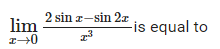Solution: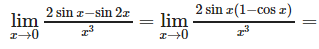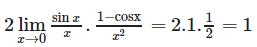QUESTION: 6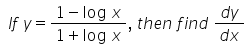Solution:

y = (1 - log x)/(1 + log x)
Applying the Quoitent rule and differential for ln x
dy / dx = [(1 - ln x)(1 / x) - (1+ ln x)( -1 / x)]/(1 - ln x)2
= [(1 - ln x + 1 + ln x)]/x(1 + ln x)2
= 2 / x(1+ln x)2

QUESTION: 7

If x = a cos φ and y = b sin φ then dy/dx =

Solution:
QUESTION: 8

If sin(x + y) = log(x + y),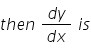:

Solution:

Given equation,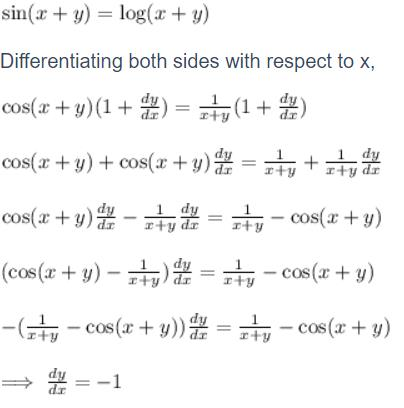QUESTION: 9

The differential coffcient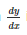of the equation yx = e(x - y) is :

Solution:

Taking log both the sides
xlogy = (x-y)loge
Differentiate it with respect to x, we get
x/y dy/dx = logy = loge - xloge dy/dx
dy/dx = (loge - logy)/(x/y + loge)
= y(1 - loge)/(x + y)

QUESTION: 10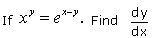Solution: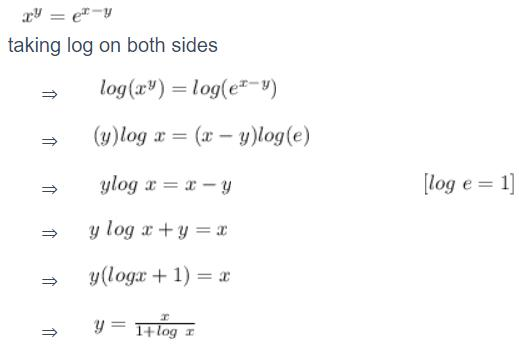On differentiating both sides with respect to x